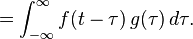# Creative Convolution – Part 2

In Part 1 we described the Impulse Response and how that can be used to characterize a room, which can be used to add a specific reverb to an audio track. In this post we’ll explore the process by which this is carried out, which is convolution.

So what is convolution? If you have two  functions f(t) and g(t), then the convolution of f and g is defined aswhich is the integral over time (represented by the symbol tau) from minus infinity to plus infinity of the product of f and a reversed and time-shifted g. So you integrate to take the common area under both functions as the reversed function slides across the first function. You can see a visualisation of this at the Wolfram Mathworld page on convolution.

This is still not an intuitive idea and it is hard to grasp what exactly convolution does. Effectively we are using the second function as a weighting function to apply to the first. This is little clearer in the discrete case. Since we are usually processing digital audio files this is what we’ll use to compute the convolution in reality. In discrete form our functions f(t) and g(t) can be represented as h[n] and x[n] with the convolution result being y[n]. Instead of integration, we are summing from minus infinity to plus infinity. The definition therefore becomes.So let’s say we have x[n] = [3,2,1] and h[n] = [2,1,3]. We’ll consider x[n] to be an input. We can break that down into a train of single impulses so

x[n] = x0[n] + x1[n] + x2[n]

We know at n=0 our input has a value of 3, at n=1 a value of 2 and at n=2 a value of 1. So we can rewrite x[n] as

x[n] = 3δ[n] + 2δ[n-1] + δ[n-2]

Now we can apply these weightings to our second function h[n]

By breaking it down into the values at a given time you can see how the weightings are applied as the function is shifted across.The youtube clip below works through this example. You can also play around with different functions at this page (java required) to see what the values are at each point in time to get a better feel for how the reversed and time-shifted signal affects the output.

What if instead of x[n] = [3,2,1] we simply had x[n] = 1? If you were to apply the convolution of x[n] and h[n] you would unsurprisingly just get h[n] back out again (this is an easy one to work out by hand using the method in the video). So h[n] is the impulse response of the filter or system, which is where it ties back into our IR reverb. By capturing the impulse response of the room (filter) and convolving that with our audio track (input) we’ll get a track with the reverb applied (output).

Here is a short example with a ukelele sample convolved with the impulse response example from part 1. The audio is normalized in both cases. (This is one thing that you need to be wary of as the output signal may experience clipping unless it is normalized).

So now that we know how convolution works, we can use Matlab or Scilab to process our audio tracks, or we can use the built in convolution function in Adobe Audition or your favourite IR reverb plugin. Since we know that convolution is just filtering one sound through another here’s where we can get creative.

The impulse response selected need not be a capture of the reverb characteristics of a room. We can also use convolution to shape sounds. In the example below a simple vocal sample is convolved with a chainsaw with interesting effects.

Convolution in this case has the effect of applying the harsh timbre of the chainsaw to the vocal. Convolution in the time domain is equivalent to multiplication in the frequency domain. What this means is that we can use convolution to emphasize certain frequencies in the audio track which are stronger in the impulse response function. There are many factors that influence the timbre of a sound but the spectral envelope is one of the primary factors so convolution is a great tool for this purpose. Dave Thomas of The Square Revolution is a musician that  makes good use of this effect. In one track, Dave told how by convolving his vocals with the timbre of a bowed violin, he is able to produce beautiful, rich, ambient vocal sounds.

Convolution can also be used in more adventurous manners. Typical convolutions involve impulse responses of only a few seconds in length. By using longer samples with more varied frequencies over time we can drastically alter a piece of music to create ambient works (if one is predisposed to aleatoric music). In the final example, the original piece is created using standard midi sounds (strings, organ, voice). By using convolution with a shorter subsection of the piece the second, more interesting piece is generated.

Update: I discovered this great article at Sound on Sound which goes into a bit more detail on how reverb works and some other ideas for creative convolution.

## 2 thoughts on “Creative Convolution – Part 2”

1.Jeffrey Smith

Thanks for the advice, and the blog honestly looks great. Just what wp design are you using?

•Matt

Thanks for the kind words. It’s currently using My Base 0.5 from myThemes

This site uses Akismet to reduce spam. Learn how your comment data is processed.